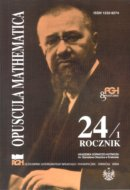Opuscula Math. 24, no. 1 (2004), 5-17

Opuscula Mathematica

# A majorization relation for a certain class of *-quivers with an orthogonality condition

O. V. Bagro
S. A. Kruglyak

Abstract. In [Kruglyak S. A., Samoĭlenko Yu. S.: On structure theorems for a family of idempotents. Ukrainskii Matematicheskii Zhurnal 50 (4), (1998) (Russian), Kruglyak S. A., Samoĭlenko Yu. S.: On the complexity of description of representations of $$*$$-algebras generated by idempotents. [in:] Proc. of the American Mathematical Society, vol. 128, 1655–1664, AMS, 2000, Kruglyak S. A.: A majorization relation for $$*$$-categories and $$*$$-wild categories. [in:] Proc. of the Fifth Intern. Conference "Symmetry in Nonlinear Math. Physics", 2004], $$*$$-algebras and $$*$$-categories over the field $$\mathbb{C}$$ of complex numbers were quasi-ordered with respect to the complexity of the structure of their $$*$$-representations with a majorization relation $$\succ$$. A notion of $$*$$-wildness was also introduced there for an algebra (a category) if the algebra majorizes the $$*$$-algebra $$C^{*}(\mathcal{F}_2)$$. In this paper, we discuss some methods for proving that an algebra is $$*$$-wild and obtain criteria for certain "standard" $$*$$-categories (ensembles with an orthogonality condition) to be $$*$$-wild.

Keywords: algebras, categories and functors, representations.

Mathematics Subject Classification: 18B30.

Full text (pdf)

• O. V. Bagro
• Institute of Mathematics of the National Academy of Sciences of Ukraine, vul. Tereshchinkivs'ka, 3, Kyiv, 01601, Ukraine
• S. A. Kruglyak
• Institute of Mathematics of the National Academy of Sciences of Ukraine, vul. Tereshchinkivs'ka, 3, Kyiv, 01601, Ukraine